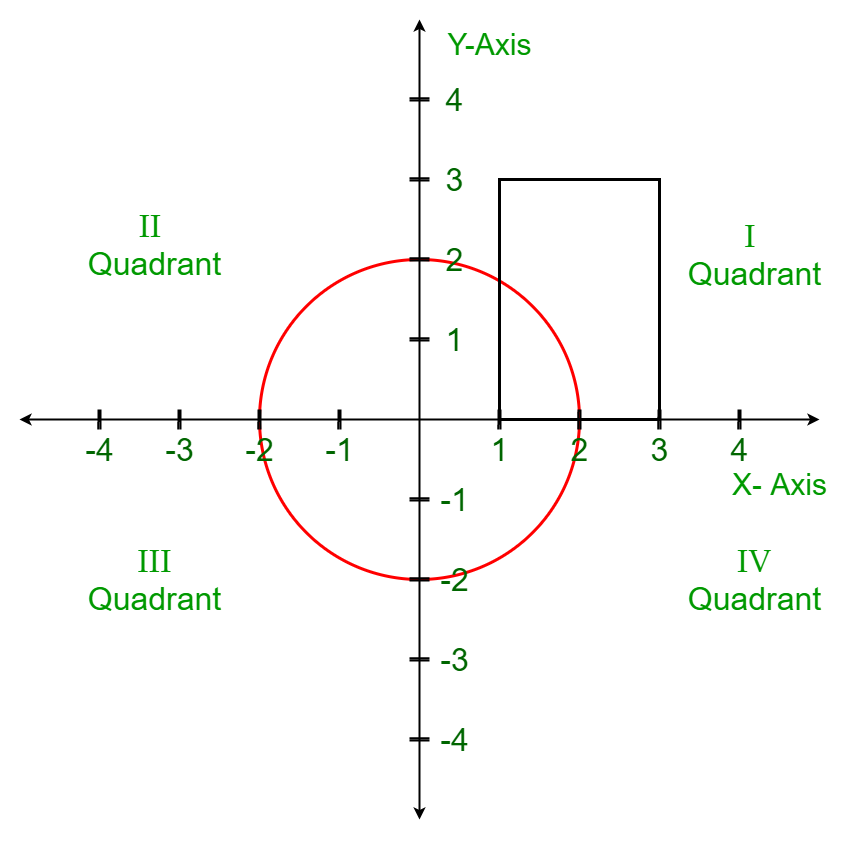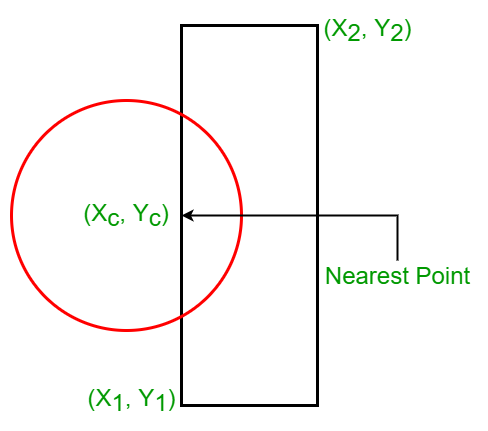# Check if any point overlaps the given Circle and Rectangle

Given two opposite diagonal points of a rectangle (X1, Y1), (X2, Y2) and the centre, radius of the circle R, (Xc, Yc), the task is to check if there exists any point P that belongs to both the circle as well as the rectangle.

Examples:

Input: R = 2, Xc = 0, Yc = 0, X1 = 1, Y1 = 0, X2 = 3, Y2 = 3
Output: true
Explanation:
Clearly, from the below illustration, the circle and the rectangle intersect.Input: R = 1, Xc = 1, Yc = 1, X1 = 3, Y1 = 3, X2 = 5, Y2 = 6
Output: false

## Recommended: Please try your approach on {IDE} first, before moving on to the solution.

Approach: The idea is to simply check if the circle and the rectangle intersect or not. There are essentially 2 possible cases when the intersection occurs.

1. Case 1: The side of the rectangle touches or intersects the circle. In order to check whether the shapes intersect, we need to find a point on or inside the rectangle that is closest to the center of the circle. If this point lies on or inside the circle, it is guaranteed that both the shapes intersect. Let the closest point be denoted by (Xn, Yn). Then the distance between the closest point and the centre of the circle can be found using sqrt((Xc- Xn)2 + (Yc- Yn)2). If this distance ≤ the radius of the circle, the two shapes intersect.
2.3. Case 2: The center of the circle lies inside the rectangle. Since the center of the circle lies inside the rectangle, the closest point will be (Xc, Yc).

On close observation, it can be observed that the point of interest only depends on the locations of (X1, Y1) and (X2, Y2) relative to (Xc, Yc). Therefore, the closest point in both the above cases can be calculated as:

1. Xn= max(X1, min(Xc, X2))
2. Yn= max(Y1, min(Yc, Y2))

Below is the implementation of the above approach:

## C++

 `// C++ implementation to check if any  ` `// point overlaps the given circle  ` `// and rectangle ` `#include ` `using` `namespace` `std; ` ` `  `// Function to check if any point  ` `// overlaps the given circle  ` `// and rectangle ` `bool` `checkOverlap(``int` `R, ``int` `Xc, ``int` `Yc, ` `                         ``int` `X1, ``int` `Y1, ` `                         ``int` `X2, ``int` `Y2) ` `{ ` ` `  `    ``// Find the nearest point on the  ` `    ``// rectangle to the center of  ` `    ``// the circle ` `    ``int` `Xn = max(X1, min(Xc, X2)); ` `    ``int` `Yn = max(Y1, min(Yc, Y2)); ` `     `  `    ``// Find the distance between the  ` `    ``// nearest point and the center  ` `    ``// of the circle ` `    ``// Distance between 2 points,  ` `    ``// (x1, y1) & (x2, y2) in  ` `    ``// 2D Euclidean space is ` `    ``// ((x1-x2)**2 + (y1-y2)**2)**0.5 ` `    ``int` `Dx = Xn - Xc; ` `    ``int` `Dy = Yn - Yc; ` `    ``return` `(Dx * Dx + Dy * Dy) <= R * R; ` `}  ` ` `  `// Driver code ` `int` `main() ` `{ ` `    ``int` `R = 1; ` `    ``int` `Xc = 0, Yc = 0; ` `    ``int` `X1 = 1, Y1 = -1; ` `    ``int` `X2 = 3, Y2 = 1; ` `     `  `    ``if``(checkOverlap(R, Xc, Yc,  ` `                       ``X1, Y1,  ` `                       ``X2, Y2)) ` `    ``{ ` `        ``cout << ``"True"` `<< endl; ` `    ``} ` `    ``else` `    ``{ ` `        ``cout << ``"False"``; ` `    ``} ` `} ` ` `  `// This code is contributed by BhupendraSingh `

## Java

 `// Java implementation to check if any  ` `// point overlaps the given circle  ` `// and rectangle ` `class` `GFG{ ` ` `  `// Function to check if any point  ` `// overlaps the given circle  ` `// and rectangle ` `static` `boolean` `checkOverlap(``int` `R, ``int` `Xc, ``int` `Yc,  ` `                                   ``int` `X1, ``int` `Y1, ` `                                   ``int` `X2, ``int` `Y2) ` `{ ` ` `  `    ``// Find the nearest point on the  ` `    ``// rectangle to the center of  ` `    ``// the circle ` `    ``int` `Xn = Math.max(X1, Math.min(Xc, X2)); ` `    ``int` `Yn = Math.max(Y1, Math.min(Yc, Y2)); ` `     `  `    ``// Find the distance between the  ` `    ``// nearest point and the center  ` `    ``// of the circle ` `    ``// Distance between 2 points,  ` `    ``// (x1, y1) & (x2, y2) in  ` `    ``// 2D Euclidean space is ` `    ``// ((x1-x2)**2 + (y1-y2)**2)**0.5 ` `    ``int` `Dx = Xn - Xc; ` `    ``int` `Dy = Yn - Yc; ` `    ``return` `(Dx * Dx + Dy * Dy) <= R * R; ` `}  ` ` `  `// Driver code ` `public` `static` `void` `main(String[] args) ` `{ ` `    ``int` `R = ``1``; ` `    ``int` `Xc = ``0``, Yc = ``0``; ` `    ``int` `X1 = ``1``, Y1 = -``1``; ` `    ``int` `X2 = ``3``, Y2 = ``1``; ` `     `  `    ``if``(checkOverlap(R, Xc, Yc,  ` `                       ``X1, Y1,  ` `                       ``X2, Y2)) ` `    ``{ ` `        ``System.out.print(``"True"` `+ ``"\n"``); ` `    ``} ` `    ``else` `    ``{ ` `        ``System.out.print(``"False"``); ` `    ``} ` `} ` `} ` ` `  `// This code is contributed by 29AjayKumar `

## Python3

 `# Python3 implementation to check if any  ` `# point overlaps the given Circle  ` `# and Rectangle ` ` `  `# Function to check if any point  ` `# overlaps the given Circle  ` `# and Rectangle ` `def` `checkOverlap(R, Xc, Yc, X1, Y1, X2, Y2):  ` ` `  `    ``# Find the nearest point on the  ` `    ``# rectangle to the center of  ` `    ``# the circle ` `    ``Xn ``=` `max``(X1, ``min``(Xc, X2)) ` `    ``Yn ``=` `max``(Y1, ``min``(Yc, Y2)) ` `     `  `    ``# Find the distance between the  ` `    ``# nearest point and the center  ` `    ``# of the circle ` `    ``# Distance between 2 points,  ` `    ``# (x1, y1) & (x2, y2) in  ` `    ``# 2D Euclidean space is ` `    ``# ((x1-x2)**2 + (y1-y2)**2)**0.5 ` `    ``Dx ``=` `Xn ``-` `Xc ` `    ``Dy ``=` `Yn ``-` `Yc ` `    ``return` `(Dx``*``*``2` `+` `Dy``*``*``2``) <``=` `R``*``*``2` ` `  `# Driver code ` `if``(__name__ ``=``=` `"__main__"``): ` `    ``R ``=` `1` `    ``Xc, Yc ``=` `0``, ``0` `    ``X1, Y1 ``=` `1``, ``-``1` `    ``X2, Y2 ``=` `3``, ``1` `     `  `    ``print``(checkOverlap(R, Xc, Yc, X1, Y1, X2, Y2)) `

## C#

 `// C# implementation to check if any  ` `// point overlaps the given circle  ` `// and rectangle ` `using` `System; ` `class` `GFG{ ` ` `  `// Function to check if any point  ` `// overlaps the given circle  ` `// and rectangle ` `static` `bool` `checkOverlap(``int` `R, ``int` `Xc, ``int` `Yc,  ` `                                ``int` `X1, ``int` `Y1, ` `                                ``int` `X2, ``int` `Y2) ` `{ ` ` `  `    ``// Find the nearest point on the  ` `    ``// rectangle to the center of  ` `    ``// the circle ` `    ``int` `Xn = Math.Max(X1,  ` `             ``Math.Min(Xc, X2)); ` `    ``int` `Yn = Math.Max(Y1,  ` `             ``Math.Min(Yc, Y2)); ` `     `  `    ``// Find the distance between the  ` `    ``// nearest point and the center  ` `    ``// of the circle ` `    ``// Distance between 2 points,  ` `    ``// (x1, y1) & (x2, y2) in  ` `    ``// 2D Euclidean space is ` `    ``// ((x1-x2)**2 + (y1-y2)**2)**0.5 ` `    ``int` `Dx = Xn - Xc; ` `    ``int` `Dy = Yn - Yc; ` `    ``return` `(Dx * Dx + Dy * Dy) <= R * R; ` `}  ` ` `  `// Driver code ` `public` `static` `void` `Main() ` `{ ` `    ``int` `R = 1; ` `    ``int` `Xc = 0, Yc = 0; ` `    ``int` `X1 = 1, Y1 = -1; ` `    ``int` `X2 = 3, Y2 = 1; ` `     `  `    ``if``(checkOverlap(R, Xc, Yc,  ` `                       ``X1, Y1,  ` `                       ``X2, Y2)) ` `    ``{ ` `        ``Console.Write(``"True"` `+ ``"\n"``); ` `    ``} ` `    ``else` `    ``{ ` `        ``Console.Write(``"False"``); ` `    ``} ` `} ` `} ` ` `  `// This code is contributed by Nidhi_biet `

Output:

```True
```

Attention reader! Don’t stop learning now. Get hold of all the important DSA concepts with the DSA Self Paced Course at a student-friendly price and become industry ready.

My Personal Notes arrow_drop_upI am currently pursuing B Tech (Hons) in Computer Science I love technology and explore new things I want to become a very good programmer I love to travel and meet new peoples

If you like GeeksforGeeks and would like to contribute, you can also write an article using contribute.geeksforgeeks.org or mail your article to contribute@geeksforgeeks.org. See your article appearing on the GeeksforGeeks main page and help other Geeks.

Please Improve this article if you find anything incorrect by clicking on the "Improve Article" button below.